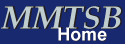Simulations in Implicit Heterogeneous Dielectric EnvironmentsObjective and Overview

This tutorial will illustrate how to setup and run a simulation of phospholamban in a heterogeneous dielectric environment as a model of a phospholipid bilayer.

Phospholamban is a membrane-bound peptide involved in cardiac muscle function. It regulates a calcium pump by switching between two conformations upon phosphorylation. Phospholamban consists of two helices, a shorter cytoplasmic one from 1-12 and a longer transmembrane helix from 20-52. The helices are connected with a flexible loop that contains the phosphorylizable residues SER16 and THR17. Experimentally observed conformations of phospholamban are roughly L-shaped with the cytoplasmic helix oriented along the membrane surface. The inhibitory conformation is believed to be fully extended with the short helix pointing away from the membrane.

### 1. Initial System Setup

Experimental conformations of phospholamban from NMR spectroscopy are available under the PDB code 1N7L. Obtain/copy the PDB file and extract the first model:
```    convpdb.pl -model 1 1N7L.pdb > 1n7l.model1.pdb
```
The heterogeneous dielectric membrane model that we will use for simulating phospholamban assumes that the membrane extends in the x-y direction, with z being the direction normal to the membrane. As you can check for yourself with VMD, the NMR structures are oriented differently with y being the direction normal to the membrane. Therefore, we need to reorient the initial model.

First, let's determine the center of mass of the transmembrane helix:
```    convpdb.pl -nsel 20-52 1n7l.model1.pdb | centerOfMass.pl
```
We can use the output from this command to translate the model so that the center of mass of the transmembrane helix coincides with the origin:
```    convpdb.pl -translate `convpdb.pl -nsel 20-52 1n7l.model1.pdb | \
centerOfMass.pl | awk '{print -\$1,-\$2,-\$3}'` \
1n7l.model1.pdb > 1n7l.model1.centered.pdb
```
By using the back quote we are using the output from one command as the input for another. If this looks too complicated, it is of course possible to do this in a manual fashion by providing the (negative) output of the first command as input to the second.

Next, we will rotate the molecule so that the transmembrane helix is aligned with the z-axis instead of the y-axis:
```    convpdb.pl -rotatex 270 1n7l.model1.centered.pdb > \
1n7l.model1.oriented.pdb
```

### 2. Generation of Effective Dielectric Profile

In order to use the heterogeneous dielectric GBMV method (HDGB) we need to provide an effective dielectric profile. The effective dielectric profile is obtained from solving the Poisson equation for a spherical probe in a layered dielectric system as a function of z.

A CHARMM script hdgb_geneps.inp is available to calculate the effective dielectric function. It has the following input parameters (to be changed at the top of the script):

 size of the probe radius (siz) 2 Å should be used epsilon in the interior (ep1) 1-3 are reasonable values epsilon in the interface (ep2) 2-20 are reasonable values epsilon in the head group/solvent region (ep3) should be 80 extent of the interior region (z1) 5-15 extent of the interface region (z2) 10-20
Good values for a DPPC phospholipid bilayer are:

ep1=2, ep2=7, ep3=80, z1=10, z2=15

Run the script to generate the profile with:
```    \$CHARMMEXEC < hdgb_geneps.inp > charmm.log
```
The result is an output file eps.dat that will be used as input to HDGB. Because many PB calculations are needed to calculate the profile, this script takes a while to complete.

This output file needs to be slightly modified before it can be used with HDGB (the first line should contain the number of data lines and δz:
```    echo `wc -l eps.dat | awk '{print \$1}'` `awk 'NR==2 {print \$1}' eps.dat` \
> eps_inp.dat
cat eps.dat >> eps_inp.dat
```

### 3. Minimize and Simulate Phospholamban with HDGB Model

We will begin with a short minimization of the phospholamban model:
```    minCHARMM.pl -par gb,hdgbprofile=eps_inp.dat,gbmvacorr=3,gbmva1=0.3255 \
-par gbmva3=1.085,gbmva4=-0.14,gbmva5=-0.15,gbmvsa=0.015 \
-par gbmvaextra="ZS 0.5 ZM 9.2 ZT 25 ST0 0.32" \
-par nocut,minsteps=100 \
-log min.log -cmd hdgb.cmd 1n7l.model1.oriented.pdb \
> plb.min.pdb
```
The HDGB method requires a few additional options with parameters as described in the HDGB papers. While the dielectric profile is taken from the eps_inp.dat input file, the profile of the non-polar interactions (modeled as gamma*SASA) is determined by the parameters ZS 0.5 ZM 9.2 ZT 25 that describe a double-switching function. These values are tuned for DPPC. For phospholipid bilayers with longer or shorter lipid tails, these values should be adjusted. In particular, ZM and ZT should be increased or decreased according to difference in width of the lipid tail region compared to DPPC. The overall magnitude of the non-polar contribution to the solvation free energy is determined by the parameter gbmvsa. This value should correspond to the desired value of gamma in bulk water.

Take a look at the file hdgb.cmd which contains the corresponding input to the CHARMM program.

We are now ready to run a short molecular dynamics run:
```    mdCHARMM.pl -par gb,hdgbprofile=eps_inp.dat,gbmvacorr=3,gbmva1=0.3255 \
-par gbmva3=1.085,gbmva4=-0.14,gbmva5=-0.15,gbmvsa=0.015 \
-par gbmvaextra="ZS 0.5 ZM 9.2 ZT 25 ST0 0.32"\
-par nocut,dynsteps=2500,dyntemp=300,lang,langfbeta=5 \
-log md1.log -trajout md1.dcd plb.min.pdb
```
In addition to the HDGB parameters used in the minimization this command will also turn on Langevin friction with a friction constant of 5/ps. Repeat the protocol above to run a second simulation with a different profile for a phospholipid bilayer where the lipid tail region is 1 Å wider and the dielectric layers have values of 1.5, 4, and 80, respectively.

### 4. Analysis of bending angle

The resulting trajectory can be analyzed with analyzeCHARMM.pl. Particularly interesting for the biological function of phospholamban is the bending angle between the two helices. This angle can be analyzed with the following command:
```    analyzeCHARMM.pl -tsel 1 15 52 -angle -pdb plb.min.pdb md1.dcd
```
The angle is defined between the center of mass of residues 1 (at the beginning of the cytoplasmic helix), 15 (at the hinge region), and 52 (at the end of the transmembrane helix. Analyze the results for both dielectric profiles and compare.

Written by M. Feig